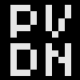# The strange beauty of the Gumowski-Mira AttractorThe world of fractals and (strange) attractors have always had my interest; mathematical formulas which expose interesting visual patterns and/or chaos when mapped to the screen. I thought I’d seen most it, but was pleasantly surprised when I recently encountered some of the organic results of the Gumowski-Mira attractor. Seemed I had some programming to do and create them myself.

The Gumowski-Mira equation was developed in 1980 at CERN by I. Gumowski and C. Mira to calculate the trajectories of sub-atomic particles. It is in fact a formula to plot a 2-dimensional dynamic system, and the main equation is as follows:

[math]f(x) = ax + frac{2(1-a) x^2 }{1 + x^2}[/math]

Using that equation, we can iterate the following formulas to calculate sequential x,y locations:

[math]x_{n+1} = by_n + f(x_n)[/math]
[math]y_{n+1} = -x_n + f(x_{n+1})[/math]

So we have two parameters, a and b (b is usually kept at 1 for the most interesting results), and to get the system going we also need some initial values for both x and y. You can set all those values in the editor below using the sliders, with some of them having a finetune-slider as well.

When you change any value, the program gives you a quick render of the results (10.000 iterations). If you want to explore more iterations, click the render (and more) button repeatedly to increase the iterations by 50.000. The color-slider is used to set the increase in blackness that each pixel will get when a point is drawn; set it to a high value if you want to quickly check how your current settings will evolve, and set it to a low value for the best images (note: the quick-render isn’t affected by this slider). Below the program are some renders I made.

[SWF]http://www.petervandernoord.nl/swf/gumowski-mira/main.swf,640,780[/SWF]## 9 thoughts on “The strange beauty of the Gumowski-Mira Attractor”

1.Cristian

Asymmetric one: http://imgur.com/kaant.png

2.tim

Very neat. What program did you use to embed the renderer?

1.Peter

The program was made in Flash.

3.quasimetric

Wow. These are beautiful. Can you share the code?

I’d like to add some CMY to the K.

1.Peter

Sounds like an interesting plan, just send me an email.

4.subblue

Very nice.

5.dr dator

awesome!